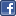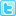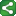Microsoft Excel Resource Center Free Macros, Formulas, Functions, Tutorials, Downloads, Add-Ins & More!
 Monte Carlo Tutorial power4XL Home
 Contents

Learning to model the problemShareThis

The real art in developing Monte Carlo models lies in translating a real-world problem into Excel equations.  This is a skill you can develop over time.  The examples later in this tutorial will give you a good start.

As a first example, look at the case of a single coin toss.  In the real world we think in terms of "heads" and "tails", but for data analysis it makes much more sense to translate these outcomes to 0 and 1.  Next you have to figure out how to convert the output generated by the RAND() function into the output of a coin toss -- i.e., take evenly distributed randomly generated numbers greater than or equal to 0 and less than 1, and translate them into two single outcomes - 0 or 1 - with a 50% probability of each.

Of course the shortcut is to use the RANDBETWEEN() function.  The following formula entered into a worksheet cell would give exactly the desired output:  "=RANDBETWEEN(0, 1)".

As your model variables increase in complexity, however, it's important to master the art of RAND() manipulation.  For the coin toss, a simple approach would be "=ROUND(RAND(),0)".  Another approach that works equally well is "=INT(RAND()*2)".

Problems with more complex input patterns require a little more creativity.  For example, suppose your problem contains a weather variable, and you know from research that in your area on a given day there is a 20% chance of rain, a 5% chance of snow, and a 75% chance of zero precipitation.

For the output, let's assume 0 = no precipitation, 1 = rain, and 2 = snow.

One approach is simply to use nested IF() statements, as illustrated in the example below.  Cell A1 contains "=RAND()".  Cell A3 contains the nested IF() equation "=IF(A1<0.05,2,IF(A1<0.25,1,0))".

Another approach to the same problem would be to use a lookup table.  Often several approaches can solve the same problem.  When one approach becomes overly complex, try using your creative capacity to find a more elegant solution.ShareThis
Prev | Contents | Next

Copyright notice:  This site and all content, including computer code and spreadsheet examples, are copyright 2006 by Fritz Dooley.  License is granted for individual users to download examples and to copy code directly into user's spreadsheets and Visual Basic for Applications files.  Users may not redistribute code in any way.  Providing hyperlinks to this web site is encouraged, but posting code and examples on other web sites is expressly forbidden.  "Microsoft" is a registered trademark of Microsoft Corporation.   Neither this web site nor Fritz Dooley is affiliated with Microsoft Corporation.